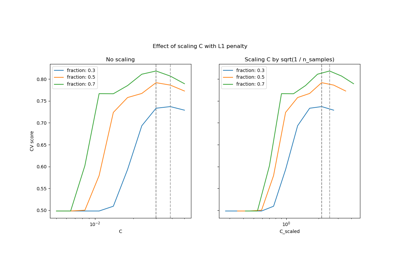# sklearn.model_selection.validation_curve¶

sklearn.model_selection.validation_curve(estimator, X, y, *, param_name, param_range, groups=None, cv=None, scoring=None, n_jobs=None, pre_dispatch='all', verbose=0, error_score=nan, fit_params=None)[source]

Validation curve.

Determine training and test scores for varying parameter values.

Compute scores for an estimator with different values of a specified parameter. This is similar to grid search with one parameter. However, this will also compute training scores and is merely a utility for plotting the results.

Read more in the User Guide.

Parameters:
estimatorobject type that implements the “fit” and “predict” methods

An object of that type which is cloned for each validation.

Xarray-like of shape (n_samples, n_features)

Training vector, where n_samples is the number of samples and n_features is the number of features.

yarray-like of shape (n_samples,) or (n_samples, n_outputs) or None

Target relative to X for classification or regression; None for unsupervised learning.

param_namestr

Name of the parameter that will be varied.

param_rangearray-like of shape (n_values,)

The values of the parameter that will be evaluated.

groupsarray-like of shape (n_samples,), default=None

Group labels for the samples used while splitting the dataset into train/test set. Only used in conjunction with a “Group” cv instance (e.g., GroupKFold).

cvint, cross-validation generator or an iterable, default=None

Determines the cross-validation splitting strategy. Possible inputs for cv are:

• None, to use the default 5-fold cross validation,

• int, to specify the number of folds in a (Stratified)KFold,

• An iterable yielding (train, test) splits as arrays of indices.

For int/None inputs, if the estimator is a classifier and y is either binary or multiclass, StratifiedKFold is used. In all other cases, KFold is used. These splitters are instantiated with shuffle=False so the splits will be the same across calls.

Refer User Guide for the various cross-validation strategies that can be used here.

Changed in version 0.22: cv default value if None changed from 3-fold to 5-fold.

scoringstr or callable, default=None

A str (see model evaluation documentation) or a scorer callable object / function with signature scorer(estimator, X, y).

n_jobsint, default=None

Number of jobs to run in parallel. Training the estimator and computing the score are parallelized over the combinations of each parameter value and each cross-validation split. None means 1 unless in a joblib.parallel_backend context. -1 means using all processors. See Glossary for more details.

pre_dispatchint or str, default=’all’

Number of predispatched jobs for parallel execution (default is all). The option can reduce the allocated memory. The str can be an expression like ‘2*n_jobs’.

verboseint, default=0

Controls the verbosity: the higher, the more messages.

error_score‘raise’ or numeric, default=np.nan

Value to assign to the score if an error occurs in estimator fitting. If set to ‘raise’, the error is raised. If a numeric value is given, FitFailedWarning is raised.

New in version 0.20.

fit_paramsdict, default=None

Parameters to pass to the fit method of the estimator.

New in version 0.24.

Returns:
train_scoresarray of shape (n_ticks, n_cv_folds)

Scores on training sets.

test_scoresarray of shape (n_ticks, n_cv_folds)

Scores on test set.

Notes

## Examples using sklearn.model_selection.validation_curve¶Plotting Validation Curves

Plotting Validation CurvesScaling the regularization parameter for SVCs

Scaling the regularization parameter for SVCs